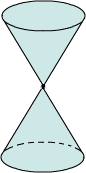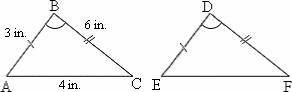## Definition Of Conic Section --gg

Conic Section deals with a group of curves obtained when a plane intersects a double cone.

Double Cone: A double cone is made up of two right circular cones placed vertex to vertex.Conic section includes circles, ellipses, parabolas, and hyperbolas.

### Solved Example on Conic Section

#### Ques: If a right circular cone is cut with a plane perpendicular to the axis of the cone, then the resultant cross section would be:A. a hyperbola
B. a circle
C. an ellipse
D. a parabola